#### Chapter 9 Triangle and its Angles R.D. Sharma Solutions for Class 9th Exercise 9.2

Exercise 9.2

1. The exterior angles, obtained on producing the base of a triangle both way are 104° and 136°. Find all the angles of the triangle.

Solution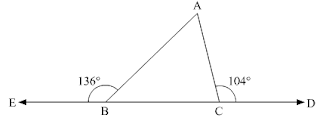∠ACD = ∠ABC + ∠BAC  [Exterior angle property]
Now,
∠ABC = 180 - 136 = 44°
∠ACB = 180 - 104 = 76°
Now,
In ΔABC,
∠A+∠ABC+∠ACB = 180  [Sum of all angles of a triangle]
⇒ ∠A + 44 + 76 = 180°
⇒ ∠A + 120 = 180°
⇒ ∠A = 180° -120°
⇒ ∠A = 60°

2. In a Δ ABC, the internal bisectors of ∠B and ∠C meet at P and the external bisectors of ∠B and ∠C meet at Q, Prove that ∠BPC + ∠BQC = 180°.

Solution3. In Fig. 9.30, the sides BC, CA and AB of a Δ ABC have been produced to D, E and F respectively. If ∠ACD = 105° and ∠EAF = 45°, find all the angles of the Δ ABC.

Solution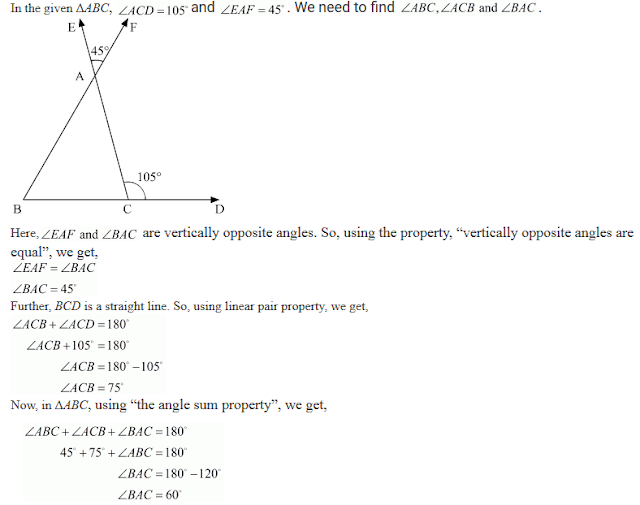4. Compute the value of x in each of the following figures: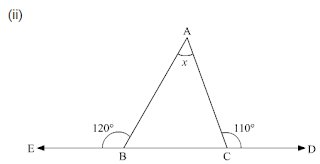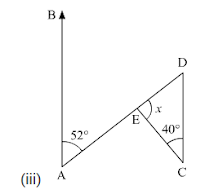Solution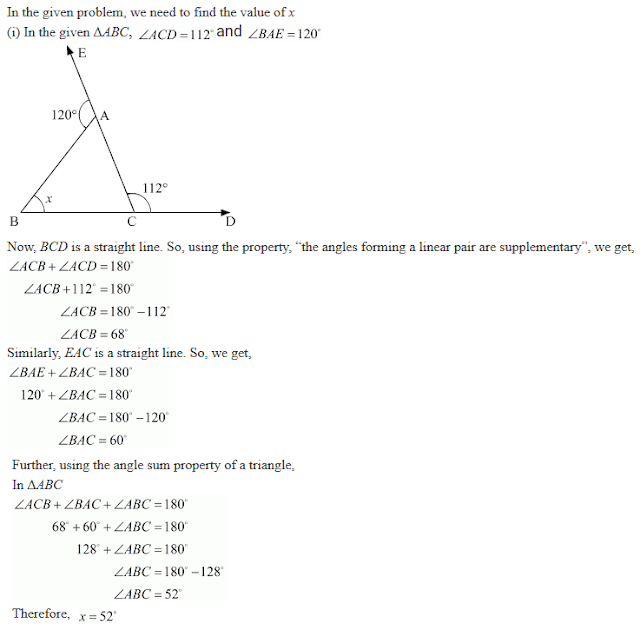5. In Fig. 9.35, AB divides ∠DAC in the ratio 1 : 3 and AB = DB. Determine the value of x.Solution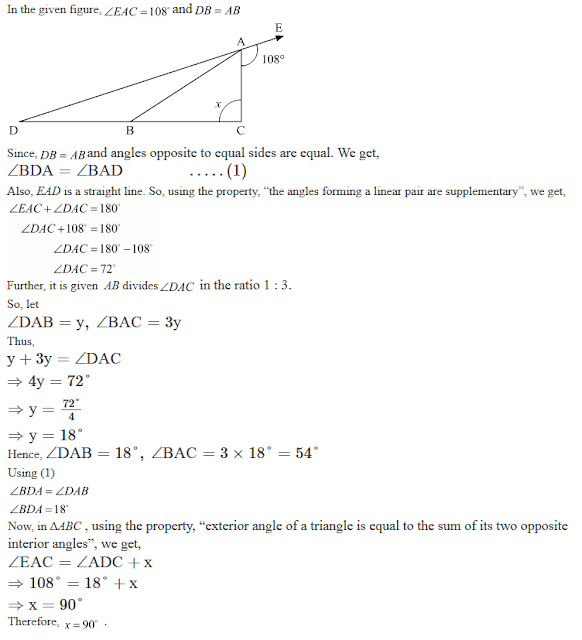6. ABC is a triangle. The bisector of the exterior angle at B and the bisector of ∠C intersect each other at D. Prove that ∠D = 1/2 ∠A.

Solution7. In Fig. 9.36, AC ⊥ CE and ∠A : ∠B : ∠C = 3 : 2 : 1, find the value of ∠ECD.Solution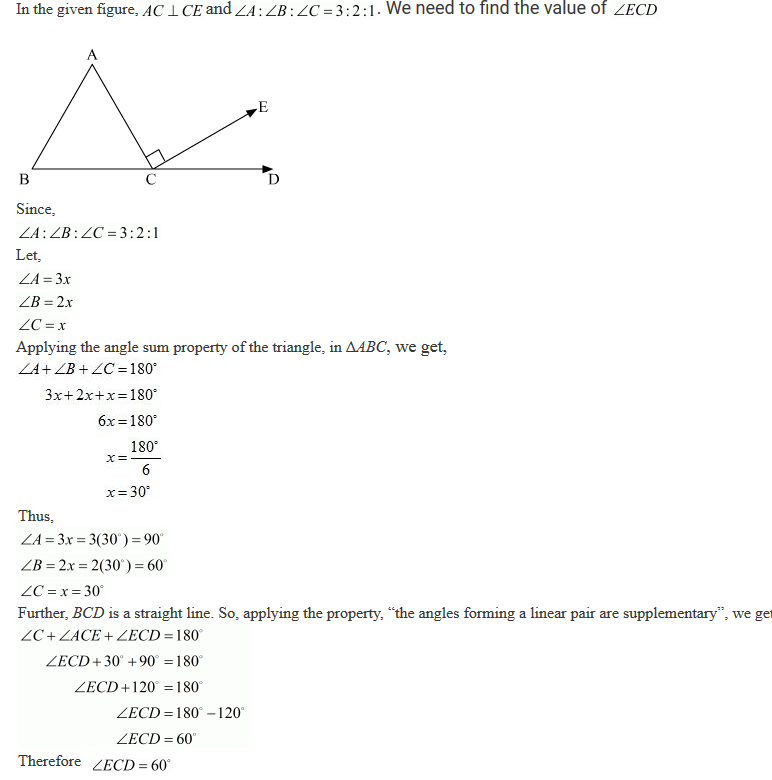8. In Fig. 9.37, AM ⊥ BC and AN is the bisector of ∠A. If ∠B = 65° and ∠C = 33°, find ∠MAN.

Solution9. In a Δ ABC, AD bisects ∠A and ∠C > ∠B. Prove that ∠ADB > ∠ADC.

Solution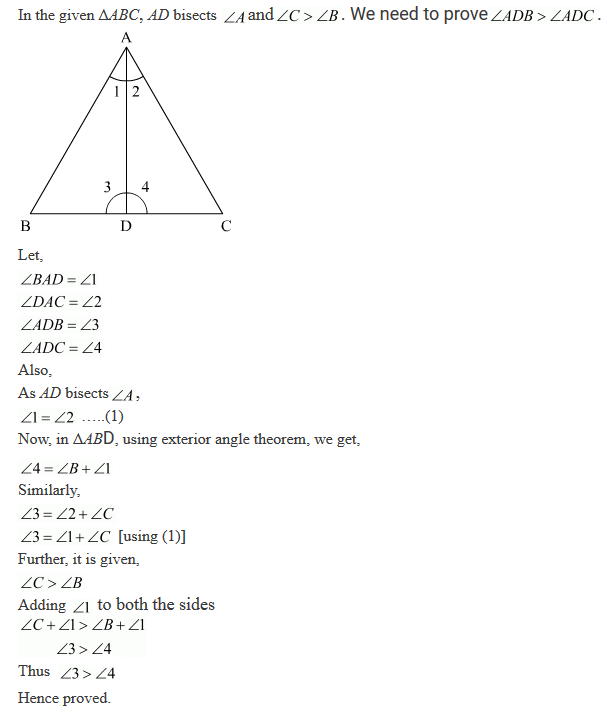10. In Δ ABC, BD⊥ AC and CE ⊥ AB. If BD and CE intersect at O, prove that ∠BOC = 180° − A.

Solution11. In a Fig. 9.38, AE bisects ∠CAD and ∠B= ∠C. Prove that AE || BC.Solution12. In Fig. 9.39, AB || DE. Find ∠ACD.Solution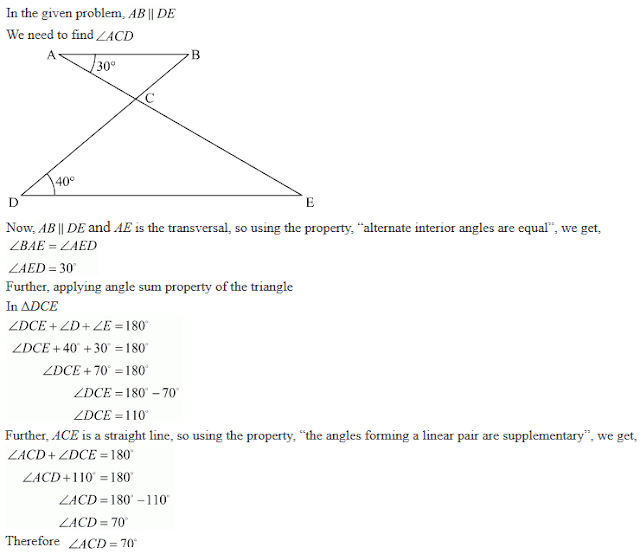13. Which of the following statements are true (T) and which are false (F):
(i) Sum of the three angles of a triangle is 180°.
(ii) A triangle can have two right angles.
(iii) All the angles of a triangle can be less than 60°
(iv) All the angles of a triangle  can be greater than 60°.
(v) All the angles of a triangle can be equal to 60°.
(vi) A triangle can have two obtuse angles.
(vii) A triangle can have at most one obtuse angles.
(viii) If one angle of a triangle is obtuse, then it cannot be a right angled triangle.
(ix) If one angle of a triangle is obtuse, then it cannot be a right angled triangle.
(x) An exterior angle of a triangle is less than either of its interior opposite angles.
(xi) An exterior angle of a triangle is equal to the sum of the two interior opposite angles.

Solution

(i) True
(ii) False
(iii) False
(iv) False
(v) True
(vi) False
(vii) True
(viii) True
(ix) False
(x) True
(xi) True

14. Fill in the blanks to make the following statements true:
(i) Sum of the angles of a triangle is _______ .
(ii) An exterior angle of a triangle is equal to the two ________ opposite angles.
(iii) An exterior angle of a triangle is always ________ than either of the interior opposite angles.
(iv) A triangle cannot have more than _______ right angles.
(v) A triangles cannot have more than _______ obtuse angles.

Solution

(i) 180°
(ii) Interior
(iii) Greater
(iv) One
(v) One